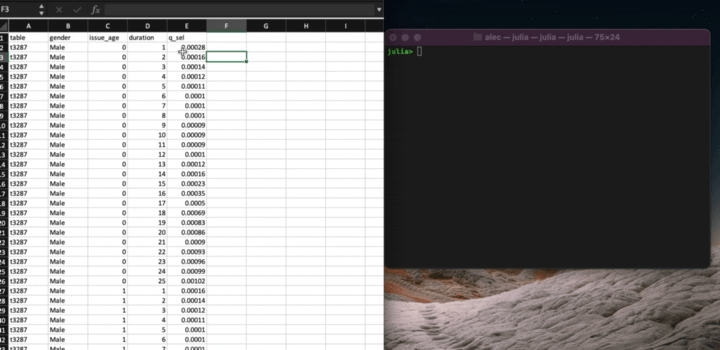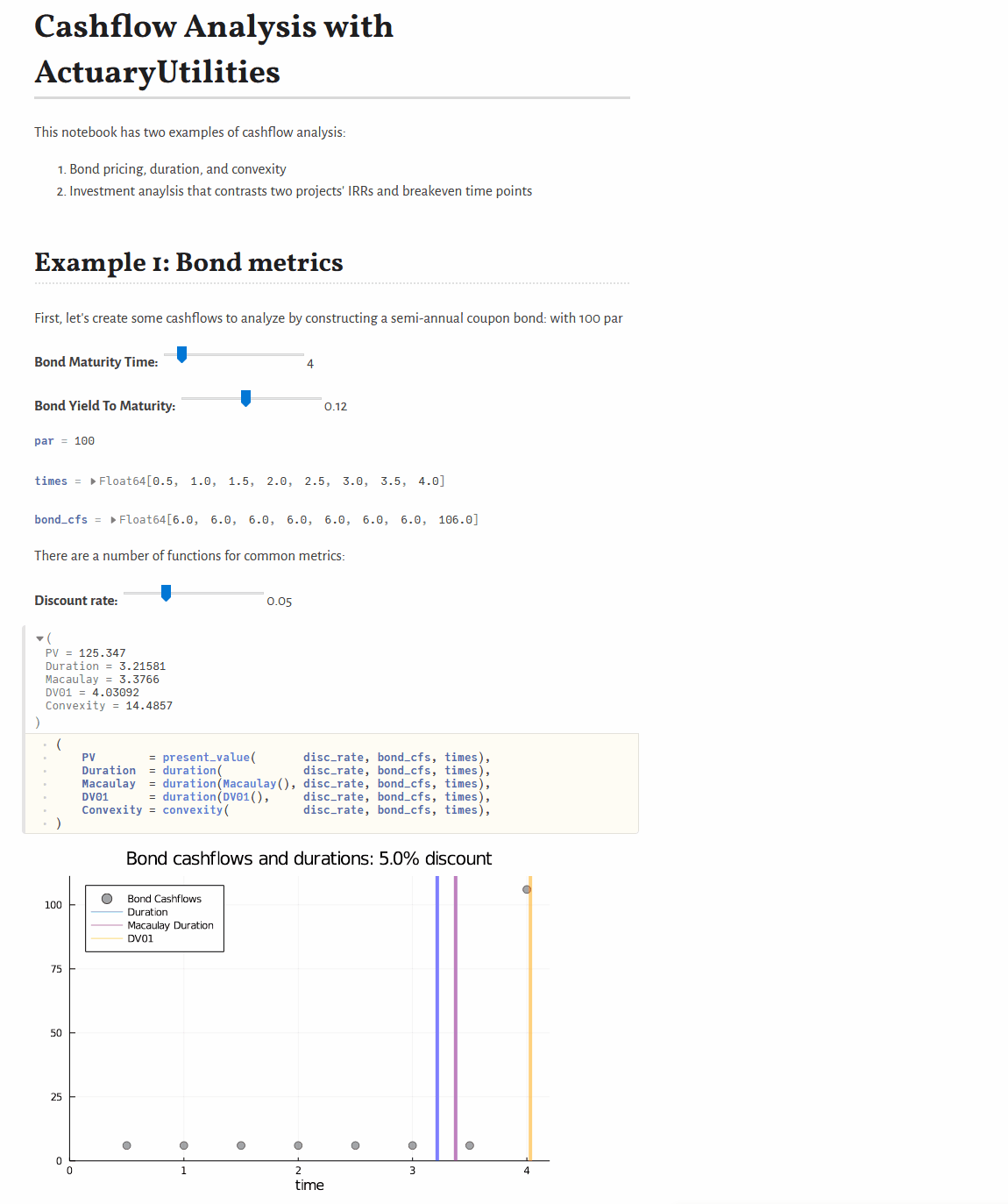## ActuaryUtilities.jl

Common functions in actuarial and financial routines
Author JuliaActuary
Popularity
7 Stars
Updated Last
2 Years Ago
Started In
March 2020

# ActuaryUtilities

## Features

A collection of common functions/manipulations used in Actuarial Calculations.

### Financial Maths

• `duration`:
• Calculate the `Macaulay`, `Modified`, or `DV01` durations for a set of cashflows
• `convexity` for price sensitivity
• Flexible interest rate options via the `Yields.jl` package.
• `internal_rate_of_return` or `irr` to calculate the IRR given cashflows (including at timepoints like Excel's `XIRR`)
• `breakeven` to calculate the breakeven time for a set of cashflows
• `accum_offset` to calculate accumulations like survivorship from a mortality vector

### Insurance mechanics

• `duration`:
• Calculate the duration given an issue date and date (a.k.a. policy duration)

### Excel Utilities

You can also copy/paste to/from Excel:• `xlcopy()` copies and parses Excel content on the clipboard
• `xlcopy(data)` will copy Julia data into your clipboard for pasting into Excel.

Also note related packages, such as XLSX.jl for working with Excel files.

## Documentation

Full documentation is available here.

## Examples

### Quickstart

```bond_cfs = [5, 5, 105]
times    = [1, 2, 3]

discount_rate = 0.03

present_value(discount_rate, cfs, times)           # 105.65
duration(Macaulay(), discount_rate, cfs, times)    #   2.86
duration(discount_rate, cfs, times)                #   2.78
convexity(discount_rate, cfs, times)               #  10.62```

### Interactive, basic cashflow analysis

See JuliaActuary.org for instructions on running this example.## Useful tips

Functions often use a mix of interest_rates, cashflows, and timepoints. When calling functions, the general order of the arguments is 1) interest rates, 2) cashflows, and 3) timepoints.